UGC NET CS 2007-Dec-Paper-2

 Question 1
A box contains six red balls and four green balls. Four balls are selected at random from the box. What is the probability that two of the selected balls are red and two are green ?
 A 3/7 B 4/7 C 5/7 D 6/7
Engineering-Mathematics       Probability
Question 1 Explanation:Question 2
The number of edges in a complete graph with ‘n’ vertices is equal to :
 A n(n-1) B n(n-1)/2 C n2 D 2n-1
Engineering-Mathematics       Graph-Theory
Question 2 Explanation:
Complete graph is an undirected graph in which each vertex is connected to every vertex, other than itself. If there are ‘n’ vertices then total edges in a complete graph is n(n-1)/2.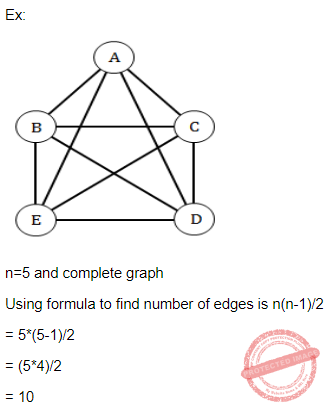Question 3
A context free grammar is :
 A type 0 B type 1 C type 2 D type 3
Theory-of-Computation       Context-Free-Grammar
Question 3 Explanation:Question 4
Let e : B^m →B^n is a group code. The minimum distance of ‘e’ is equal to :
 A the maximum weight of a non zero code word B the minimum weight of a non zero code word C m D n
 Question 5
Consider a Moore machine M whose digraph is :Then L(M), the language accepted by the machine M, is the set of all strings having :
 A two or more b’s. B three or more b’s. C two or more a’s. D three or more a’s.
Theory-of-Computation       Moore-and-Mealy-Machine
Question 5 Explanation:
In the given state diagram it can be clearly noticed that the minimum string that is accepted by the moore machine M is "bb". So the language can contain 0 or more number of a's and 2 or more number of b's.
 Question 6
A WFF that is equivalent to the WFF x=>y is :
 A y=> x B ~ y=> x C ~ y=> ~ x D y=> ~ x
Engineering-Mathematics       Propositional-Logic
Question 6 Explanation: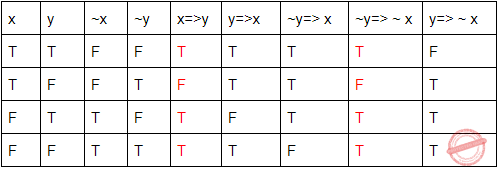Question 7
Simplified form of Boolean expression xy+(~x)z+yz is :
 A xy+(~x)z. B (~x)y+(~x)z. C (~x)y+xz. D xy+xz.
Digital-Logic-Design       Boolean-Expression
Question 7 Explanation:Question 8
In order to build a MOD-18 counter, the minimum number of flip flops needed is equal to :
 A 18 B 9 C 5 D 4
Digital-Logic-Design       Sequential-Circuits
Question 8 Explanation:
Mod value of a counter is the number of unique states.
Let K = Number of states =18.
Number of flip-flops is the smallest number which is greater than or equal to Log K ( base 2).
Log 18 = 4.1.
L=5>=4.1.
So number of flip-flops needed is 5.
 Question 9
The dual of the switching function F=x+yz is given by :
 A x+yz B x(y+z) C (~x)+(~y)(~z) D (~x)((~y)+(~z))
Digital-Logic-Design       Dual-Function
Question 9 Explanation:
The dual of switching function means it changes AND to OR and OR to AND.
given x+yz.
x.(y+z)
 Question 10
Amongst the logic families DTL, TTL, ECL and CMOS, the family with the least power dissipation is :
 A CMOS B DTL C TTL D ECL
Question 10 Explanation:
Emitter Coupled Logic (ECL):
The storage time is eliminated as the transistors are used in difference amplifier mode and are never driven into saturation.
1. Fastest among all logic families
2. Lowest propagation delay.
Complementary metal oxide semiconductor(CMOS):
The power dissipation is usually 10nW per gate depending upon the power supply voltage, output load etc.
1. Lowest power dissipation 2. Excellent noise immunity 3. High packing density 4. Wide range of supply voltage 5. Highest fan out among all logic families Transistor Transistor Logic:
→ TTL devices consume substantially more power than equivalent CMOS devices at rest, but power consumption does not increase with clock speed as rapidly as for CMOS devices.
 Question 11
What cannot replace ‘?’ in the following C-code to print all odd numbers less than 100 ?
for (i=1; ? ; i=i+2)
printf(“%d\n”,i);
 A i≤100 B i≤101 C i<100 D i<101
Programming       Control-Statement
Question 11 Explanation:
The given constraint in question, we have to print odd numbers in less than 100.
So, the condition should not be greater than 100 but it is equal to 100 acceptable.
for (i=1; i<=100 ; i=i+2)
printf(“%d\n”,i);
 Question 12
Consider the following linked list:Which of the following piece of code will insert the node pointed to by q at the end of the list ?
 A for (p=list; p !=NULL; p=p →next); p=q; B for (p=list; p !=NULL; p=p →next); p →next=q; C for (p=list; p →next!=NULL; p=p→next); p=q; D for (p=list; p →next !=NULL; p=p →next); p →next=q;
Question 12 Explanation:
→ n order to add a new node to the end of the list, first we need to traverse the entire list.
→ To the traverse the list, we will take one pointer.
→ We will traverse the list till the end by moving the pointer to next node.
→ The pointer will point to the last node and from there the pointer next address will point to the new node.
Option D will provide the above steps.
Q
 Question 13
Which of the following is a valid C code to print character ‘A’ to ‘C’ ?
 A x=’A’; switch(x) { case ‘A’=printf (“%d\n”, x); .... case ‘C’=printf (“%d\n”, x); } B x=’A’; switch(x) { case ‘A’<=x <=’C’; printf (“%d\n”, x); } C x=’A’; switch(x) { case ‘A’ : printf (“%d\n”, x); break; case ‘B’ : printf (“%d\n”, x); break; case ‘C’ : printf (“%d\n”, x); break; } D x=’A’; switch(x) { case ‘A’=printf (“%d\n”, x); case ‘B’=printf (“%d\n”, x); case ‘C’=printf (“%d\n”, x); } E None of the above
Programming       Switch-Case
Question 13 Explanation:
→ The options A,B and D are syntactically incorrect.
→ Option C will print only one character "A"
→ All four options are invalid according to the question.
 Question 14
Which of the following is not true in C++ ?
 A “Private” elements of a base class are not accessible by members of its derived class. B “Protected” elements of base class are not accessible by members of its derived class. C When base class access specified is “Public”, public elements of the base class become public members of its derived class. D When base class access specified is “Public”, protected elements of a base class become protected members of its derived class.
Programming-in-c++       Properties
Question 14 Explanation:
TRUE: “Private” elements of a base class are not accessible by members of its derived class.
FALSE: “Protected” elements of base class are not accessible by members of its derived class.
TRUE: When base class access specified is “Public”, public elements of the base class become public members of its derived class.
TRUE: When base class access specified is “Public”, protected elements of a base class become protected members of its derived class.
 Question 15
Which of the following is true of constructor function in C++ ?
 A A class must have at least one constructor. B A constructor is a unique function which cannot be overloaded. C A constructor function must be invoked with the object name. D A constructor function is automatically invoked when an object is created.
Programming-in-c++       Constructor-Destructor
Question 15 Explanation:
FALSE: A class must have at least one constructor.
FALSE: A constructor is a unique function which cannot be overloaded.
FALSE: A constructor function must be invoked with the object name.
TRUE: A constructor function is automatically invoked when an object is created.
 Question 16
A primary key for an entity is :
 A a candidate key B any attribute C a unique attribute D a superkey
Database-Management-System       Candidate-key
Question 16 Explanation:
A primary key for an entity is a unique attribute. No primary key should have NULL values.
 Question 17
Aggregate functions in SQL are :
 A GREATEST, LEAST and ABS B SUM, COUNT and AVG C UPPER, LOWER and LENGTH D SQRT, POWER and MOD
Database-Management-System       SQL
Question 17 Explanation:
Aggregate functions in SQL are SUM,COUNT,AVG,MIN and MAX.
The COUNT() function returns the number of rows that matches a specified criteria.
The AVG() function returns the average value of a numeric column.
The SUM() function returns the total sum of a numeric column.
 Question 18
If a relation is in 2NF and 3NF forms then :
 A no non-prime attribute is functionally dependent on other non-prime attributes B no non-prime attribute is functionally dependent on prime attributes C all attributes are functionally independent D prime attribute is functionally independent of all non-prime attributes
Database-Management-System       Normalization
Question 18 Explanation:
2NF: A relation is said to be in 2NF if there is no partial dependency which means a relation is said to be in 2NF if there is no Prime key attribute which is functionally defining a non-key prime attribute. But a prime attribute can functionally determine a prime attribute.
Example: Let us consider a relation R(A, B, C, D) and let’s take ABC is the primary key of the given relation R then A, B, C are the prime attributes and D is a non-key prime attribute.
Now for 2NF, B → D should not exist but B → A can exist.
3NF: A relation is said to be in 3NF if no transitive dependency exist in the relation i.e. either the LHS of a functional dependency should be a super key or the RHS of a functional dependency should be a Prime attribute.
Option(A) is correct it means the the LHS will always be a super key or both LHS or RHS of a functional dependency will be a prime key attribute. In both the cases the relation will be in 3NF and when the relation will be in 3NF it will automatically be in 2NF.
Option (B) is incorrect because according to this if there could be a case when in a functional dependency its LHS will not have prime key attribute and RHS will also not be a prime key attribute. So in that case the relation can't be in 3NF. so this option is not correct.
Option(C) is incorrect, if all attributes will be functionally independent then the conditions of being in 2NF and 3NF will not be satisfied. SSo this option is incorrect.
 Question 19
The end of an SQL command is denoted by :
 A an end-of-line character B an ‘enter-key’ marker C entering F4 key D a semicolon (;)
Database-Management-System       SQL
Question 19 Explanation:
A semicolon (;) tells SQL*Plus that you want to run the command. Type the semicolon at the end of the last line of the command.
 Question 20
Consider the query : SELECT student_name FROM students WHERE class_name=(SELECT class_name FROM students WHERE math_marks=100);
what will be the output ?
 A the list of names of students with 100 marks in mathematics B the names of all students of all classes in which at least one student has 100 marks in mathematics C the names of all students in all classes having 100 marks in mathematics D the names and class of all students whose marks in mathematics is 100
Database-Management-System       SQL
Question 20 Explanation: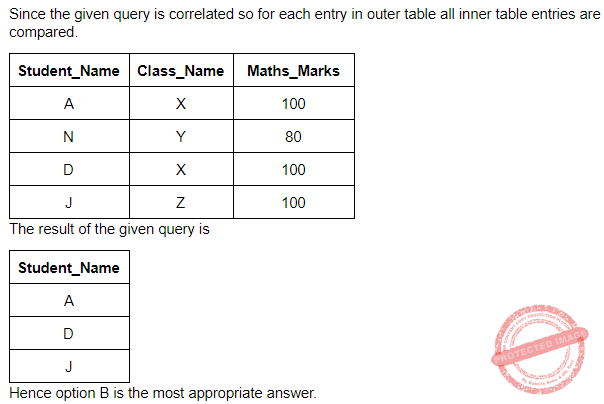Question 21
Consider a rooted tree in which every node has at least three children. What is the minimum number of nodes at level i (i > 0) of the tree ? Assume that the root is at level 0 :
 A 3i B 3i C 3 D 3i + 1
Data-Structures       Trees
Question 21 Explanation:
From the given question,
Given condition is every node has at least three children.
the root node is present at level 0, here only one is present(30)
at level 1, three nodes are present based upon the condition( 31 nodes)
at level 2, nine nodes will be present (32 nodes ) [Each node has at least three children]
and so on the at level "i", 3i nodes will be present.
 Question 22
Which of the following data structure is used to implement recursion ?
 A Arrays B Stacks C Queues D Linked lists
Data-Structures       Queues-and-Stacks
Question 22 Explanation:
→ Stack is used to implement recursion. It uses Last in first out procedure.
→ Recursion is one the application of stack. It supports many applications.
Applications:
1. Expression Evaluation
2. Expression Conversion
3. Syntax Parsing
4. Backtracking
5. Parenthesis Checking
6. String Reversal
 Question 23
The height of a binary tree with ‘n’ nodes, in the worst case is :
 A O(log n) B O(n) C Ω(n log n) D Ω (n2)
Data-Structures       Binary-Trees
Question 23 Explanation:
The height of a binary tree with ‘n’ nodes, in the worst case is O(n). Sometimes, it will get left skewed/ right skewed based on given input.Question 24
An example of a file extension is :
 A text B pict C mp3 D web
Question 24 Explanation:
→ Text,pict and web is not file extensions.
→ mp3 is one of the extensions of audio files.
 Question 25
The performance of a file system depends upon the cache hit rate. If it takes 1 msec to satisfy a request from the cache but 10 msec to satisfy a request if a disk read is needed, then the mean time (ms) required for a hit rate ‘h’ is given by :
 A 1 B h + 10 (1 - h) C (1 - h) + 10 h D 10
Computer-Organization       Cache
Question 25 Explanation:
Mean time = (Cache hit rate)*(cache access time) + (cache miss rate)(memory access time)
= Mean time = (h*1)+(1-h)(10)
= Mean time = h+(1-h)(10)
 Question 26
The best known example of a MAN is :
 A Ethernet B Cable Television C FDDI D IEEE 802.3
Computer-Networks       Area-of-Networks
Question 26 Explanation:
Option-A: It is ruled out because it is a cable to get internet.
Option-B: Cable television is one of the example of MAN.
Option-C: It is ruled out because it is a cable to get internet.
Option-D: IEEE 802.3 it is actual name for ethernet.
 Question 27
In a broadcast network, a layer that is often thin or non-existent is :
 A network layer B transport layer C presentation layer D application layer
Computer-Networks       TCP/IP-Layers
Question 27 Explanation:
In a broadcast network, a layer that is often thin or non-existent is network layer.
 Question 28
The maximum data rate of binary signals on a noiseless 3 KHz channel is :
 A 3000 bps B 6000 bps C 9000 bps D 12,000 bps
Question 28 Explanation:
Given data,
-- Bandwidth=3 KHz =3000 bps
-- Discrete levels(L)=2
-- Noise channel=?
Step-1: To find noiseless channel using Nyquist Bit Rate.
Nyquist Bit Rate = 2 * Bandwidth * log2(L)
= 2*3000*log22
= 2*3000*1
= 6000 bps
 Question 29
For pure ALOHA, the maximum channel utilization is :
 A 100% B 50% C 36% D 18%
Computer-Networks       Access-Control-Methods
Question 29 Explanation: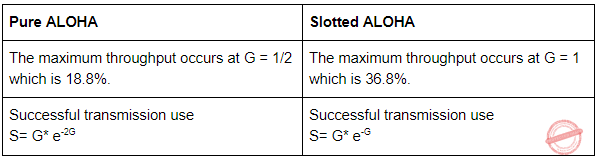Question 30
An example of an adaptive routing algorithm is :
 A distance vector routing B flooding C selective flooding D shortest path routing
Computer-Networks       Routing
Question 30 Explanation:
→ Adaptive routing algorithms which change their routing decisions whenever network topology or traffic load changes. The changes in routing decisions are reflected in the topology as well as traffic of the network.
→ An example of an adaptive routing algorithm is distance vector routing.
 Question 31
In a two pass compiler, during the first pass :
 A user defined address symbols are correlated with their binary equivalent B the syntax of the statement is checked and mistakes, if any, are listed C object program is generated D semantic of the source program is elucidated.
Compiler-Design       Compilers
Question 31 Explanation:
→ In a two pass compiler, during the first pass, user defined address symbols are correlated with their binary equivalent.
→ During first pass in two pass compilers contain lexical,syntactic,semantic and intermediate code generator are in front end.
→ During second pass in two pass compilers contain code optimization and code generator are in back end.
 Question 32
A single instruction in an assembly language program contains :
 A one micro operation B one macro operation C one instruction to be completed in a single pulse D one machine code instruction
Compiler-Design       Compilers
Question 32 Explanation:
A single instruction in an assembly language program contains one macro operation.
 Question 33
Absolute loader demands that the programmer needs to know the :
 A start address of the available main memory B total size of the program C actual address of the data location D absolute values of the operands used
Question 33 Explanation:
Absolute loader demands that the programmer needs to know the start address of the available main memory.
 Question 34
Top-down parsers are predictive parsers, because :
 A next tokens are predicted. B length of the parse tree is predicted beforehand C lowest node in the parse tree is predicted. D next lower level of the parse tree is predicted.
Compiler-Design       Parsers
Question 34 Explanation:
→ Top-down parsers are predictive parsers, because next tokens are predicted.
→ Predictive parser is a recursive descent parser, which has the capability to predict which production is to be used to replace the input string.
→ The predictive parser does not suffer from backtracking.
→ Predictive parsing uses a stack and a parsing table to parse the input and generate a parse tree.
 Question 35
In the context of compiler design, “reduction in strength” refers to :
 A code optimization obtained by the use of cheaper machine instructions B reduction in accuracy of the output C reduction in the range of values of input variables D reduction in efficiency of the program
Compiler-Design       Code-Optimization
Question 35 Explanation:
In the context of compiler design, “reduction in strength” refers to code optimization obtained by the use of cheaper machine instructions.
 Question 36
How many states can a process be in ?
 A 2 B 3 C 4 D 5
Operating-Systems       Process-State-Transition-Diagram
Question 36 Explanation:
Total 5 states in process.
1. New
3. Running
4. Waiting
5. Terminate
 Question 37
A program has five virtual pages, numbered from 0 to 4. If the pages are referenced in the order 012301401234, with three page frames, the total number of page faults with FIFO will be equal to :
 A 0 B 4 C 6 D 9
Operating-Systems       Memory-Management
Question 37 Explanation: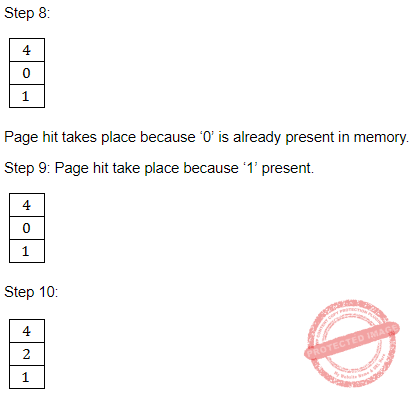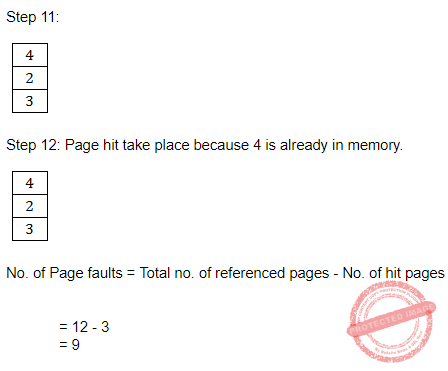Question 38
Average process size = s bytes. Each page entry requires e bytes. The optimum page size is given by :
 A √(se) B √(2se) C s D e
Operating-Systems       Memory-Management
Question 38 Explanation: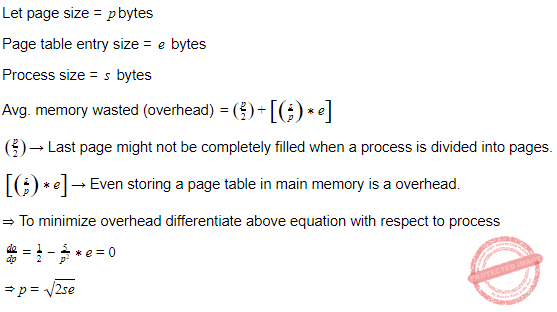Question 39
An example of a directory found in most UNIX system is :
 A usr. B waitpid. C brk. D unmap.
Operating-Systems       UNIX-Operating-System
Question 39 Explanation:
The “usr” directory is default location of user home directories.
Sub directories:
1. /usr/bin
2. /usr/include
3. /usr/lib
4. /usr/spool
5. /usr/tmp
 Question 40
The aging algorithm with a = 0.5 is used to predict run times. The previous four runs from oldest to most recent are 40, 20, 20, and 15 msec. The prediction for the next time will be :
 A 15 msec B 25 msec C 39 msec D 40 msec E None of the above
Question 40 Explanation: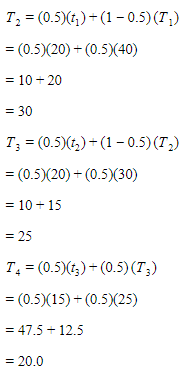Question 41
A major defect in waterfall model in software development is that :
 A the documentation is difficult B a blunder at any stage can be disastrous C a trial version is available only at the end of the project D the maintenance of the software is difficult
Software-Engineering       Waterfall-model
Question 41 Explanation:
A major defect in waterfall model in software development is that a trial version is available only at the end of the project.
 Question 42
Function point metric of a software also depends on the :
 A number of function needed B number of final users of the software C number of external inputs and outputs D time required for one set of output from a set of input data
Software-Engineering       Software-design
Question 42 Explanation:
Function point metric of a software also depends on the number of external inputs and outputs.
 Question 43
An error message produced by an interactive system should have :
 A always the error code B the list of mistakes done by the user displayed C a non-judgmental approach D the past records of the occurrence of the same mistake
Question 43 Explanation:
An error message produced by an interactive system should have the list of mistakes done by the user displayed.
 Question 44
System development cost estimation with use-cases is problematic because :
 A of paucity of examples B the data can be totally incorrect C the expertise and resource available are not used D the problem is being over simplified
Software-Engineering       Software-Development
Question 44 Explanation:
System development cost estimation with use-cases is problematic because the data can be totally incorrect.
 Question 45
The approach to software testing is to design test cases to :
 A break the software B understand the software C analyse the design of sub processes in the software D analyze the output of the software
Software-Engineering       Software-testing
Question 45 Explanation:
The approach to software testing is to design test cases to break the software.
 Question 46
Given a parallel algorithm A with computation time t, if parallel algorithm A performs m computational operation, then p processors can execute algorithm A in time given by :
 A t/p B mt/p C t+(m-t)/p D (m-t)/p
Question 46 Explanation:
According to Brent’s theorem, Given A, a parallel algorithm with computation time t, if parallel algorithm A performs m computational operations, then processors can execute algorithm A in time [t + {(m – t) / p}].
 Question 47
With reference to implementation of different association mining algorithms, identify the correct statement :
 A The FP growth method was usually better than the best implementation of the apriori algorithm B Apriori algorithm is usually better than CHARM C Apriori algorithm is good when the support required is low D At very low support the number of frequent items becomes less
Question 47 Explanation:
TRUE: The FP growth method was usually better than the best implementation of the apriori algorithm
FALSE: Apriori algorithm is usually better than CHARM
FLASE: Apriori algorithm is good when the support required is low
FALSE: At very low support the number of frequent items becomes less
 Question 48
Consider a typical mobile communication system. If 840 frequencies are available, how many can be used in a given cell ?
 A 140 B 120 C 84 D 60
Question 48 Explanation: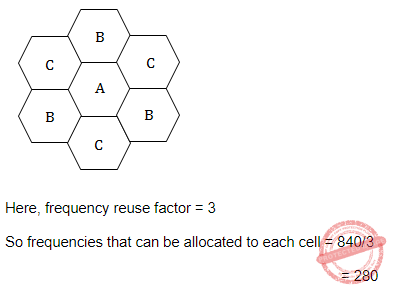Question 49
Identify the incorrect statement :
 A The internet has evolved into phenomenally successful e-commerce engine B e-business is synonymous with e-commerce C The e-commerce model B2C did not begin with billboardware D The e-commerce model G2C began with billboardware
Question 49 Explanation:
TRUE: The internet has evolved into phenomenally successful e-commerce engine
FALSE: e-business is synonymous with e-commerce
TRUE: The e-commerce model B2C did not begin with billboardware
TRUE: The e-commerce model G2C began with billboardware
 Question 50
Identify the incorrect statement :
 A ATM provides both real time and non-real time service B ATM provides faster packet switching than X.25 C ATM was developed as part of the work on broadband ISDN D ATM does not have application in Non-ISDN environments where very high data rates are required
Question 50 Explanation:
TRUE: ATM provides both real time and non-real time service
TRUE: ATM provides faster packet switching than X.25
TRUE: ATM was developed as part of the work on broadband ISDN TRUE: ATM does not have application in Non-ISDN environments where very high data rates are required
There are 50 questions to complete.
PHP Code Snippets Powered By : XYZScripts.com